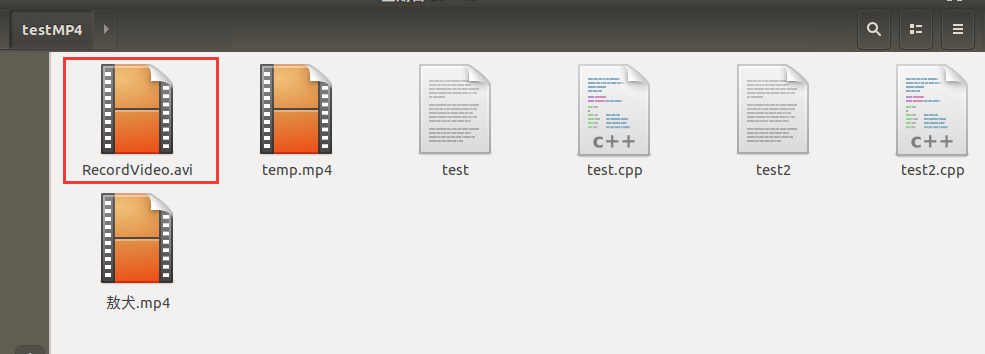WHCSRL 技术网

# Ubuntu 18下OpenCV3.4的安装与使用示例

## Ubuntu 18下OpenCV3.4的安装与使用示例

VirtualBox虚拟机Ubuntu 18.04系统
OpenCV版本：3.4.14

## 一、认识 OpenCV

OpenCV的应用领域包括:

• 2D和3D功能工具包
• 运动估计
• 面部识别系统
• 手势识别
• 人机交互
• 移动机器人
• 动作理解
• 物体识别
• 分割和识别
• 实体影像立体视觉:来自两个摄像机的深度感知
• 运动中的结构(SFM)
• 运动跟踪
• 增强现实

• 提升(Boosting)
• 决策树学习
• 梯度提升树
• 期望最大化算法
• k最近邻算法
• 朴素贝叶斯分类器
• 人工神经网络
• 随机森林
• 支持向量机(SVM)
• 深层神经网络(DNN)

## 二、安装 OpenCV

### 1. 下载 OpenCV 3.4.11 数据包

OpenCV下载地址：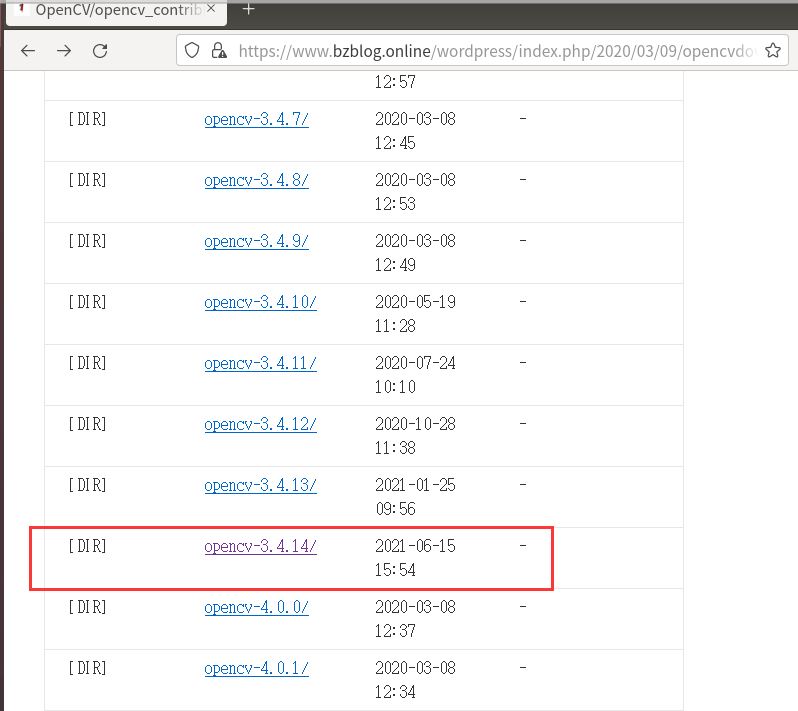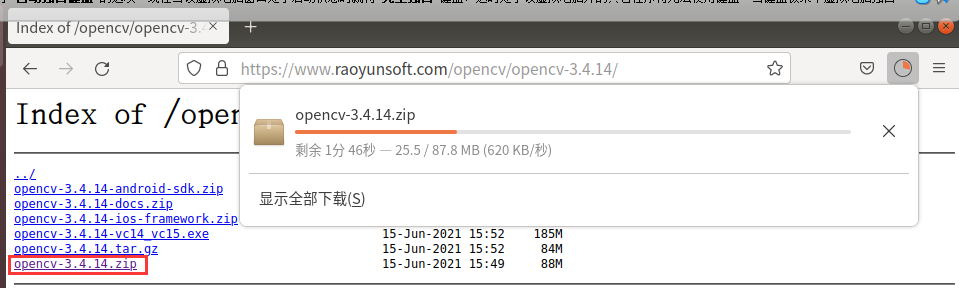Ubuntu中游览器的默认下载位置是名为“下载”的文件夹，如果不能通过cd进入可以选择右键文件夹选择终端打开来解决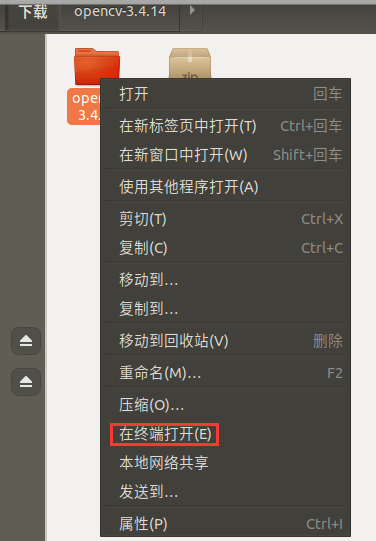### 2. 解压安装

``````unzip opencv-3.4.14.zip
```1```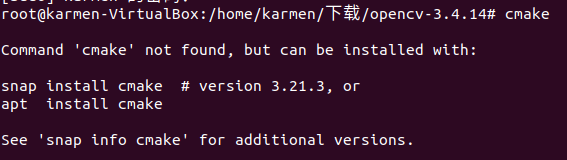``````apt-get install cmake
```1```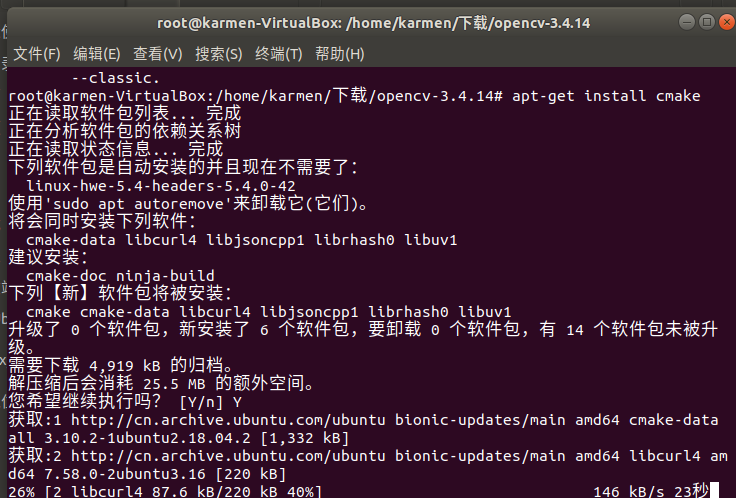``````sudo apt-get update
```1```

``````sudo apt-get install build-essential libgtk2.0-dev libavcodec-dev libavformat-dev libjpeg.dev libtiff5.dev libswscale-dev libjasper-dev
```1```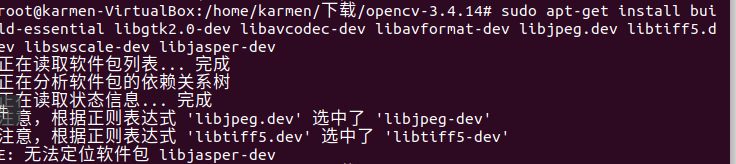``````sudo add-apt-repository "deb http://security.ubuntu.com/ubuntu xenial-security main"
sudo apt update
sudo apt install libjasper1 libjasper-dev
```1234```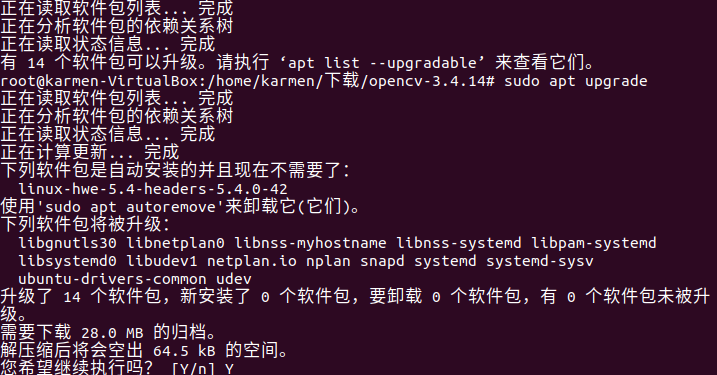``````mkdir build
cd build
```12```

``````cmake -D CMAKE_BUILD_TYPE=Release -D CMAKE_INSTALL_PREFIX=/usr/local ..
```1```

``````sudo make -j4
```1```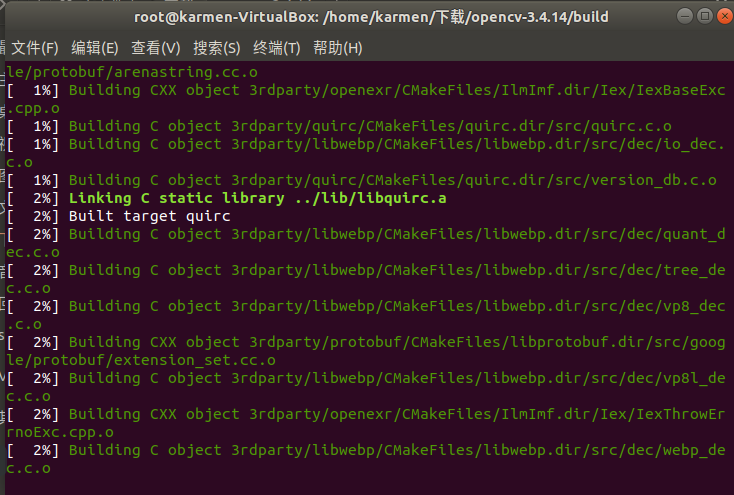``````sudo make install
```1```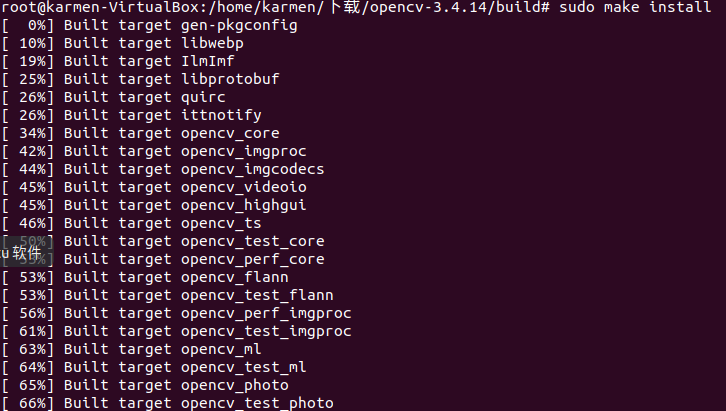### 3. 配置环境

``````sudo gedit /etc/ld.so.conf.d/opencv.conf
```1```

``````/usr/local/lib
```1```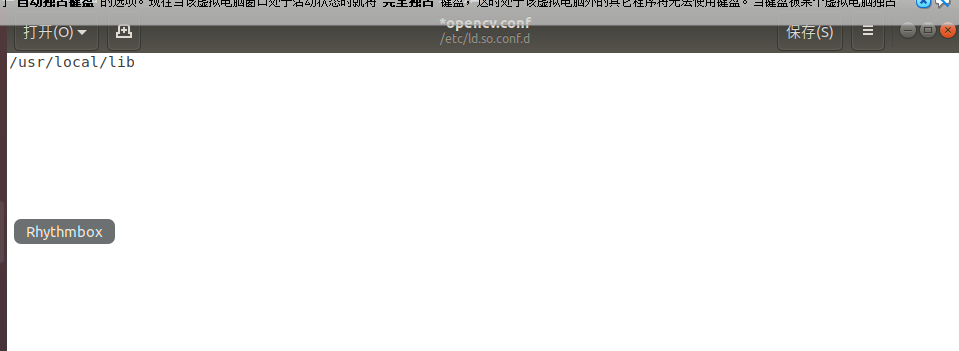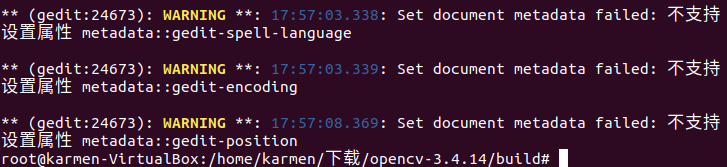``````sudo ldconfig
```1```

``````sudo gedit /etc/bash.bashrc
```1```

``````PKG_CONFIG_PATH=\$PKG_CONFIG_PATH:/usr/local/lib/pkgconfig
export PKG_CONFIG_PATH
```12```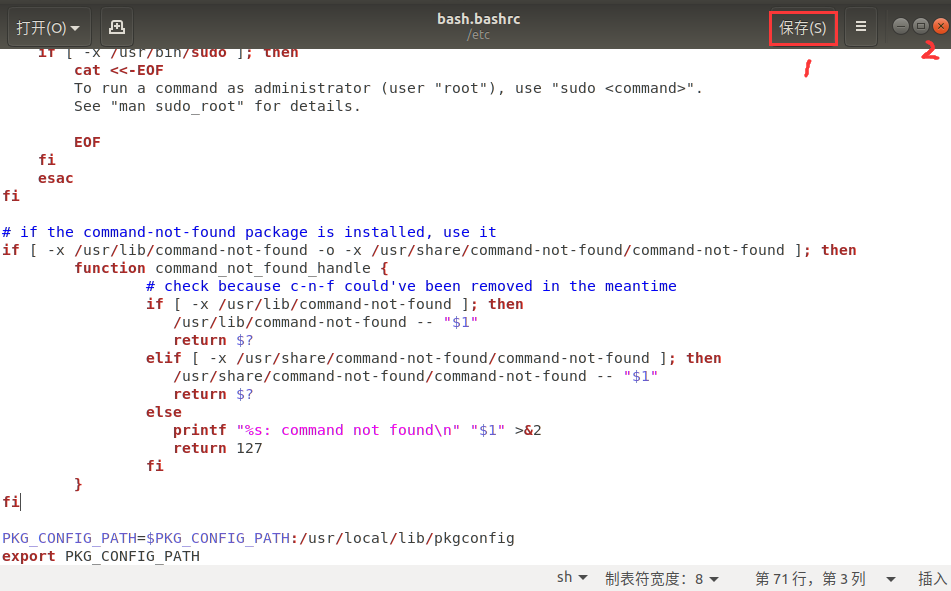``````source /etc/bash.bashrc
sudo updatedb
```12```

``````pkg-config --modversion opencv
```1```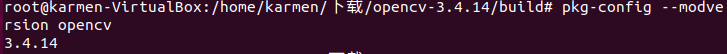## 三、编译图片

#### 1. 创建文件

``````gedit test.cpp
```1```

``````#include <opencv2/highgui.hpp>
#include <opencv2/opencv.hpp>
using namespace cv;
using namespace std;
int main(int argc, char** argv)
{
CvPoint center;
double scale = -3;

IplImage* image = cvLoadImage("temp.jpg");
argc == 2? cvLoadImage(argv) : 0;

cvShowImage("Image", image);
if (!image) return -1; 	center = cvPoint(image->width / 2, image->height / 2);
for (int i = 0;i<image->height;i++)
for (int j = 0;j<image->width;j++) {
double dx = (double)(j - center.x) / center.x;
double dy = (double)(i - center.y) / center.y;
double weight = exp((dx*dx + dy*dy)*scale);
uchar* ptr = &CV_IMAGE_ELEM(image, uchar, i, j * 3);
ptr = cvRound(ptr * weight);
ptr = cvRound(ptr * weight);
ptr = cvRound(ptr * weight);
}

Mat src;Mat dst;
src = cvarrToMat(image);
cv::imwrite("test.png", src);

cvNamedWindow("test",1);  	imshow("test", src);
cvWaitKey();
return 0;
}
```123456789101112131415161718192021222324252627282930313233```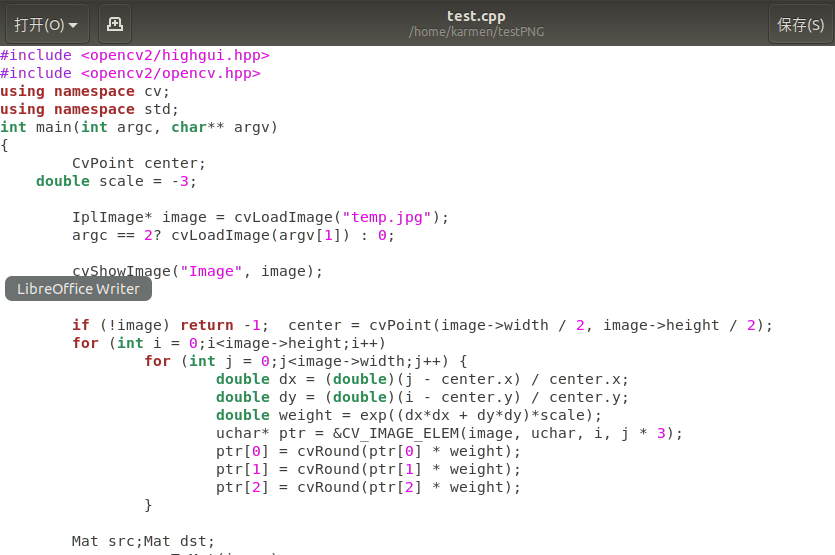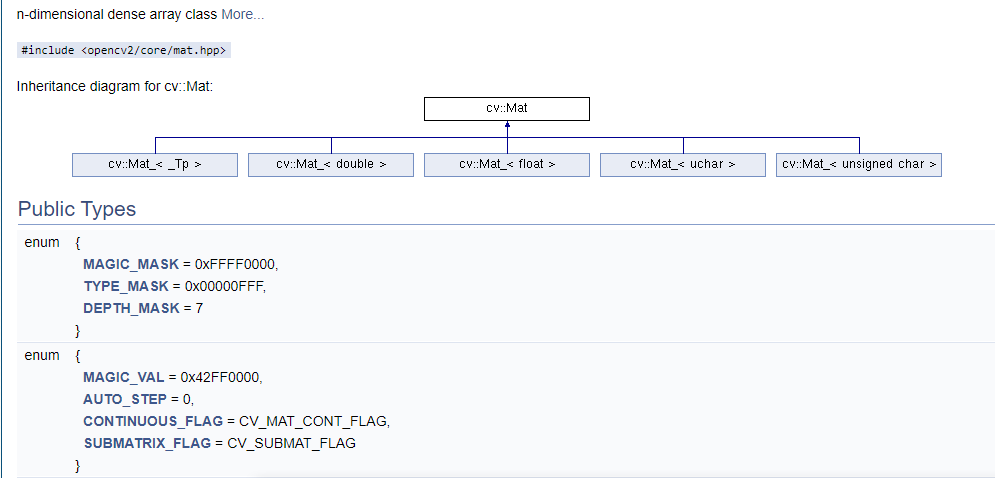### 2. 编译

OpenCV的矩阵是用Mat这个对象来存储的，并且提供了一些函数操作矩阵的属性。关于Mat数据类型的更多知识，请在OpenCV官网或者在CSDN上搜索。

``````gcc test.cpp -o test `pkg-config --cflags --libs opencv`
```1```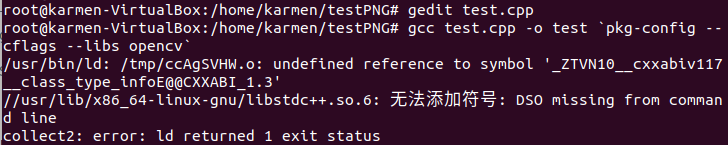``````g++ test.cpp -o test `pkg-config --cflags --libs opencv`
```1```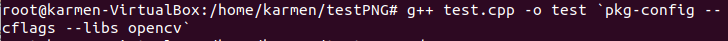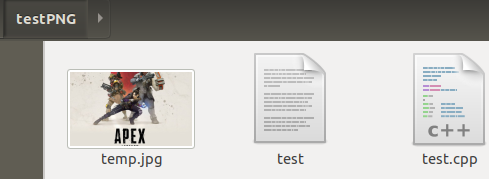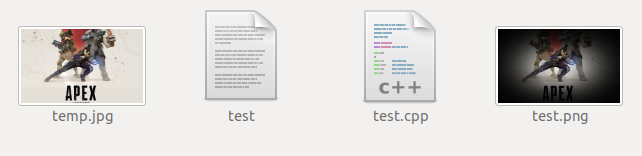## 四、编译视频

### 1. 环境配置：

• 在虚拟机里难免要使用内置或外置的摄像头
• 比如在做ROS By Example例子就要使用usbcam
• 比如开发Opencv，使用摄像头捕抓图像
• 要使用USB，就需要安装扩展Oracle_VM_VirtualBox_Extension_Pack

1. 下载Oracle_VM_VirtualBox_Extension_Pack
点击进入下载页面，选择对应版本，因为本人使用的6以上的版本所以这里选择6.1.0_RC1，然后下载下图所标注的任意一个拓展包。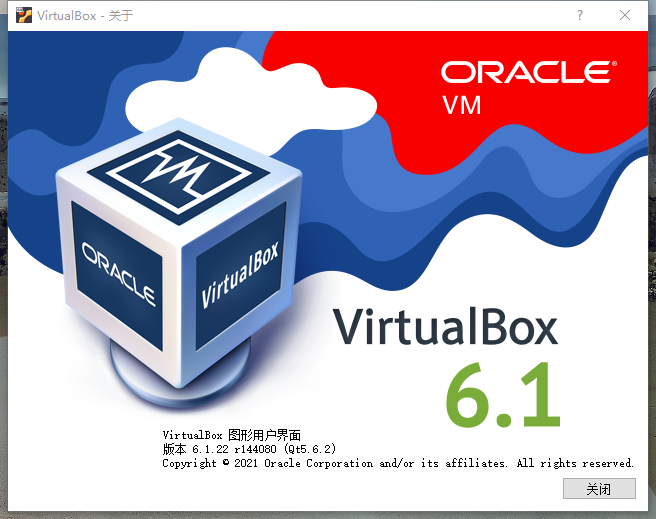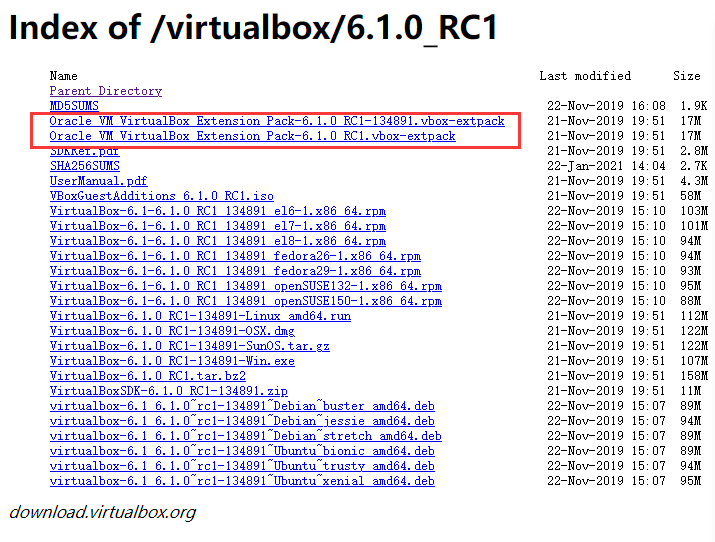2. 安装到virtualbox, 在`virtualbox管理器`👉`管理`👉`全局设定`👉`扩展`，并在右上角的添加扩展,选择拓展包所在位置，确定。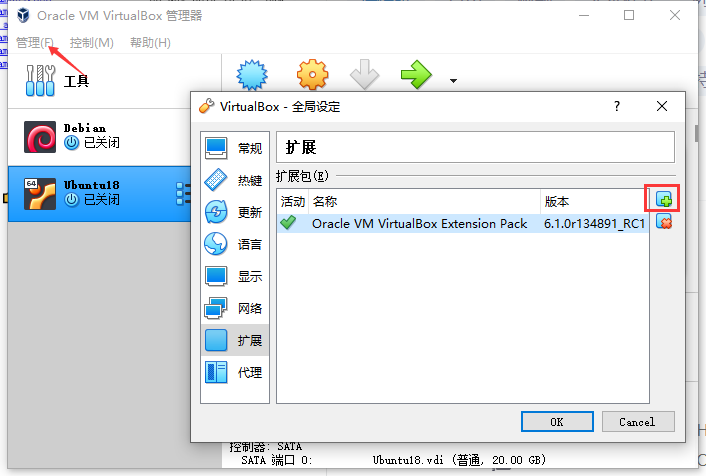3. 启用摄像头
安装完成后，启动虚拟机。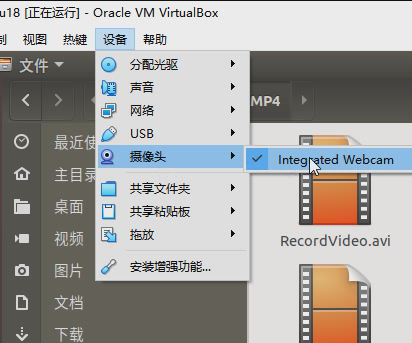``````ls /dev/video*
```1```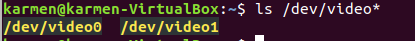``````sudo apt-get install -y //cheese安装 cheese
cheese                  //调用 cheese
cheese -d /dev/video1   //调用指定的摄像头
```123```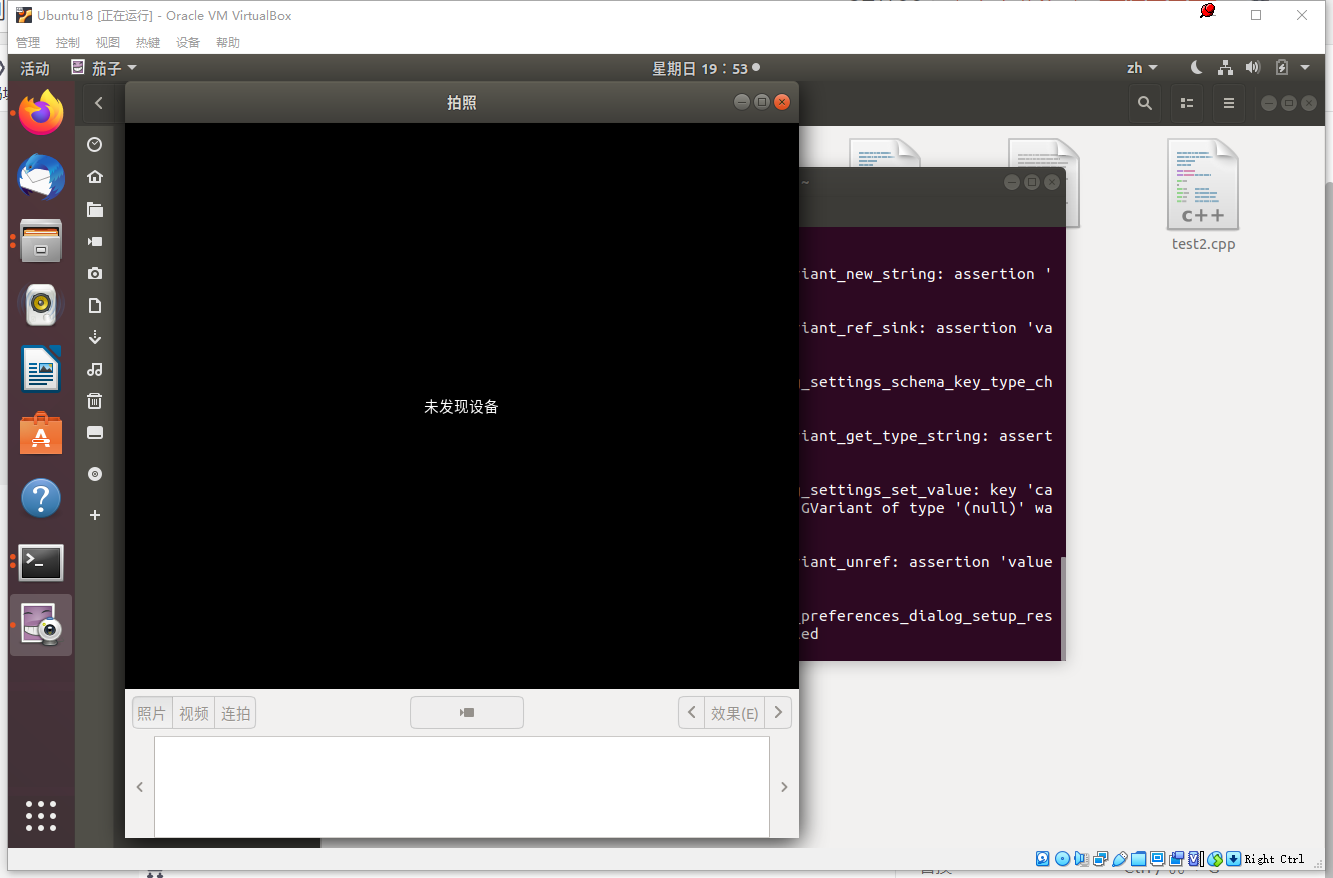``````安装 xawtv
sudo apt-get install -y xawtv

xawtv

xawtv -d /dev/video1
```12345678```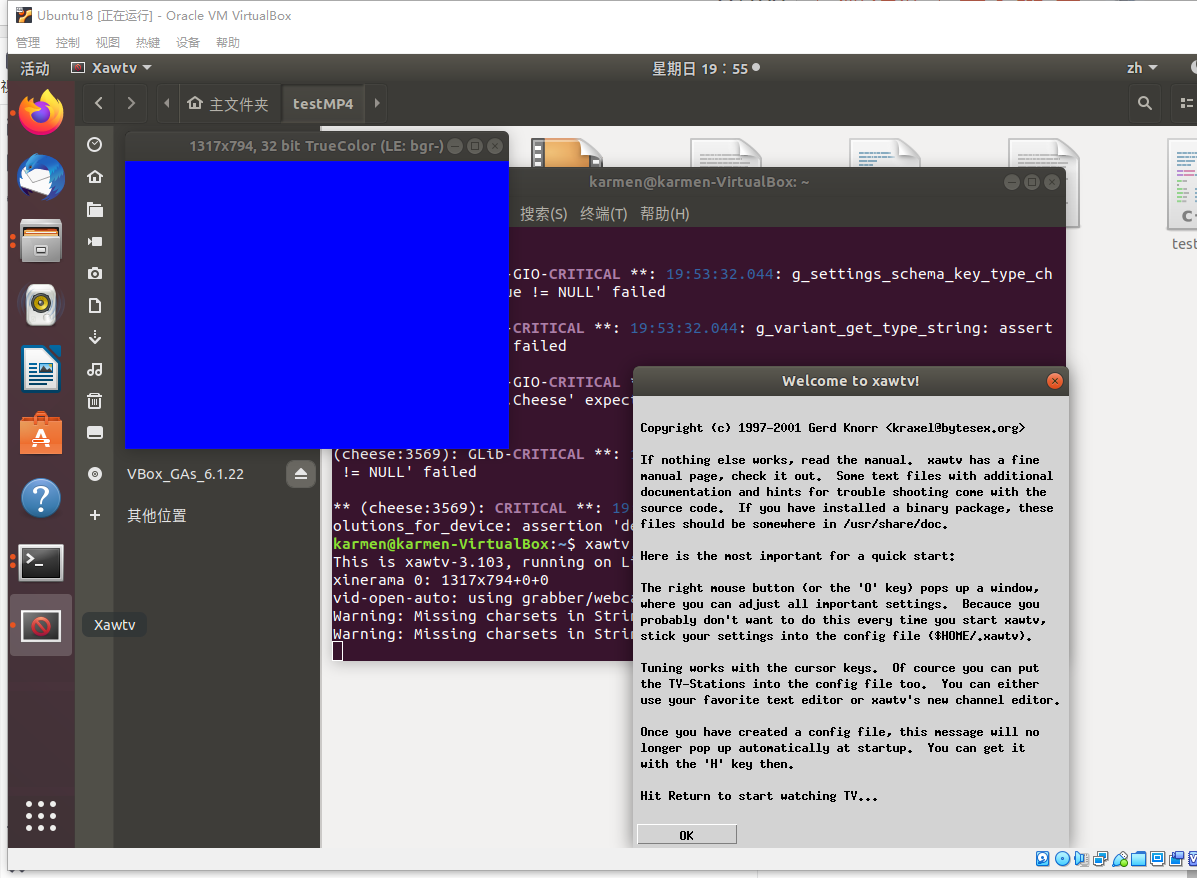### 2. 播放视频

``````#include <iostream>
#include <opencv2/core/core.hpp>
#include <opencv2/highgui/highgui.hpp>
using namespace std;
using namespace cv;

int main(int argc, char* argv[])
{
VideoCapture capture;
//capture.open(0);   //打开摄像头
capture.open("temp.mp4");   //打开视频
if (!capture.isOpened())
{
cout << "video not open." << endl;
return 1;
}
double rate = capture.get(CV_CAP_PROP_FPS);     	//获取当前视频帧率
//当前视频帧
Mat frame;
//每一帧之间的延时
int delay = 1000/ rate;      	//与视频的帧率相对应
while (1)
{
{
cout << "no video frame" << endl;
break;
}
imshow("video", frame);
//引入延时
//也可通过按键停止
int k = waitKey(30);
// 实现按键暂停和退出视频播放功能
if (k == 27)
break;
else if (k == 32)
{
while (waitKey(0) != 32)
waitKey(0);
}
}
//关闭视频，手动调用析构函数（非必须）
capture.release();
return 0;
}
```123456789101112131415161718192021222324252627282930313233343536373839404142434445```### 3. 录制视频

``````#include<iostream>
#include <opencv2/opencv.hpp>
#include<opencv2/core/core.hpp>
#include<opencv2/highgui/highgui.hpp>
using namespace cv;
using namespace std;

int main()
{
//打开电脑摄像头
VideoCapture cap(0);
if (!cap.isOpened())
{
cout << "error" << endl;
waitKey(0);
return 0;
}

//获得cap的分辨率
int w = static_cast<int>(cap.get(CV_CAP_PROP_FRAME_WIDTH));
int h = static_cast<int>(cap.get(CV_CAP_PROP_FRAME_HEIGHT));
Size videoSize(w, h);
VideoWriter writer("RecordVideo.avi", CV_FOURCC('M', 'J', 'P', 'G'), 25, videoSize);

Mat frame;
int key;//记录键盘按键
char startOrStop = 1;//0  开始录制视频； 1 结束录制视频
char flag = 0;//正在录制标志 0-不在录制； 1-正在录制

while (1)
{
cap >> frame;
key = waitKey(100);
if (key == 32)//按下空格开始录制、暂停录制   可以来回切换
{
startOrStop = 1 - startOrStop;
if (startOrStop == 0)
{
flag = 1;
}
}
if (key == 27)//按下ESC退出整个程序，保存视频文件到磁盘
{
break;
}

if (startOrStop == 0 && flag==1)
{
writer << frame;
cout << "recording" << endl;
}
else if (startOrStop == 1)
{
flag = 0;
cout << "end recording" << endl;

}
imshow("picture", frame);
}
cap.release();
writer.release();
destroyAllWindows();
return 0;
}
```1234567891011121314151617181920212223242526272829303132333435363738394041424344454647484950515253545556575859606162636465```

``````g++ test2.cpp -o test2 `pkg-config --cflags --libs opencv`
./test2
```12```